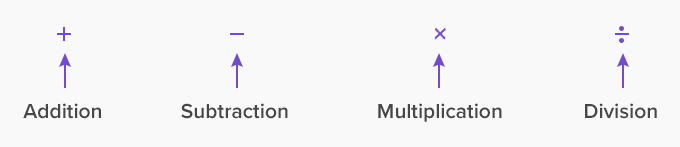Algebra - Definition with Examples

The Complete K-5 Math Learning Program Built for Your Child

• 30 Million Kids

Loved by kids and parent worldwide

• 50,000 Schools

Trusted by teachers across schools

• Comprehensive Curriculum

Aligned to Common Core

What is Algebra?

The arithmetic operations of addition, subtraction, multiplication, and division help us solve mathematical problems. Algebra deals with these concepts and can be considered as generalized arithmetic.A variable is an important concept of algebra. It can be an object or a letter that represents a number of things. We use variables to represent unknowns, to represent quantities that vary, and to generalize properties.

The letters of the English alphabet, as well as Roman symbols, are commonly used for variables.

An equation is a mathematical sentence with an equal sign.

Example: 3 + 5 = 8

Inequality is a mathematical sentence that contains symbols <, >, ≤, ≥, or  ≠.

Example: 4x + 7y ≥ 15

Equations and inequalities arise from everyday life situations.

Example: Tina wants to buy pencils and pens for \$15. Each pencil costs \$1, and the pen costs \$2 each. If x represents the number of pencils and y stands for pens, then

x + 2y ≤ 15

The algebraic thinking involved in correlating and translating the situations into equations and then solving them to find the solution for the everyday problems develops mathematical reasoning in children.

Patterns are other tools that boost children’s mathematical thinking processes. A pattern is a regular arrangement of numbers, objects or shapes. There are mainly two types of patterns:

Increasing or decreasing pattern:

12, 24, 36, 48,...

108, 102, 96, 90,...Repeating pattern:Example 1: Mathew gets paid \$2 per hour for his part-time work in a farm. If he works for 10 hours a week, how much money will he make?

For an hour he gets \$2, for two hours \$4, for 3 hours \$6, and so on.

Let us tabulate this sequence and find the earning for the week.

 No. of hours Pay (\$) 1 2 2 4 3 6 4 8 5 10 6 12

Analyzing the pattern helps us find the amount that Mathew gets paid for any number of hours of work. The pattern in the sequence is that the pay is double the number of hours that he works. Therefore, for 10 hours of work, he will make \$20.

Example 2: A library has two plans,

Plan 1: You can register for \$5 and rent any book for \$2,

Plan 2: Without registration, you can rent any book for \$3.

If Michelle is renting 7 books which plan would be beneficial?

The cost of 1 book in plan 1 is \$7. Let us represent this as an ordered pair (1, \$7) where the first number represents the number of books and the second number represents the cost. Then the cost for the first 7 books can be written as (1, \$7), (2, \$9), (3, \$11), (4, \$13), (5, \$15), (6, \$17), and (7, \$19).  Similarly, for plan 2, the cost can be calculated as (1, \$3), (2, \$6), (3, \$9), (4, \$12), (5, \$15), (6, \$18), and (7, \$21). Comparing the costs, plan 2 is beneficial.

Won Numerous Awards & Honors